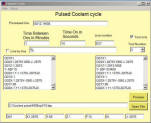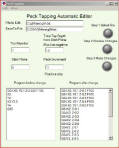Distance across Corners

These calculators are  for reference. JanProducts gives no warranty, express or implied, as to the accuracy, reliability and completeness of any information, formulae or calculations provided through the use of these calculators and does not accept any liability for loss or damage of whatsoever nature, which may be attributable to the reliance on and use of these calculators.

Distance across corners calculator

Calculates square and hex
with dimension given across flats

Enter distance across flat (i.e 1/2 hex=.5)

Distance across flats

Square: Hexagon:

Distance across corners:
Area of section:

Distance accross corners instructions
This can be used to calculate to check what diameter of tube the stock will
fit in. It can be also used to calculate the weight of a bar by multiplying
the area x length x weight/unit.

Enter the measurement accross the flat of the square or the hexagon.

Select the radio button for square or hex

Hit the Calc button to perform calculation

The result will give the measurement accross the corners and the area of
that section.aaaaaaaaaaaaiii